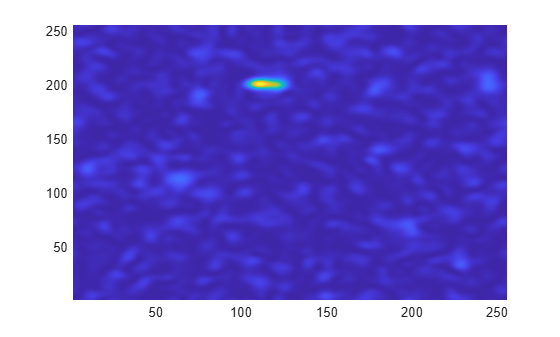# Two-Dimensional CWT of Noisy Pattern

This example shows how to detect a pattern in a noisy image using the 2-D continuous wavelet transform (CWT). The example uses both isotropic (non-directional) and anisotropic (directional) wavelets. The isotropic wavelet is not sensitive to the orientation of the feature, while the directional wavelet is.

Use the isotropic (non-directional) Mexican hat wavelet, also known as the Ricker wavelet, and the anisotropic (directional) Morlet wavelet. Demonstrate that the real-valued Mexican hat wavelet does not depend on the angle.

```Y = zeros(32,32); Y(16,16) = 1; cwtmexh = cwtft2(Y,'wavelet','mexh','scales',1,... 'angles',[0 pi/2]); surf(real(cwtmexh.cfs(:,:,1,1,1))); shading interp; title('Angle = 0 Radians');```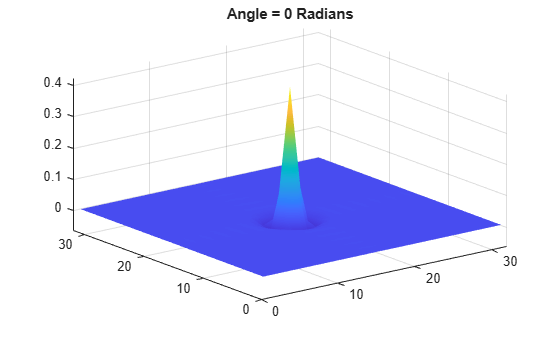Extract the wavelet corresponding to an angle of $\pi$/2 radians. The wavelet is isotropic and therefore does not differentiate oriented features in data.

```surf(real(cwtmexh.cfs(:,:,1,1,2))); shading interp; title('Angle = pi/2 Radians');```Repeat the preceding steps for the complex-valued Morlet wavelet. The Morlet wavelet has a larger spatial support than the Mexican hat wavelet, therefore this example uses a larger matrix. The wavelet is complex-valued, so the modulus is plotted.

```Y = zeros(64,64); Y(32,32) = 1; cwtmorl = cwtft2(Y,'wavelet','morl','scales',1,... 'angles',[0 pi/2]); surf(abs(cwtmorl.cfs(:,:,1,1,1))); shading interp; title('Angle = 0 Radians');```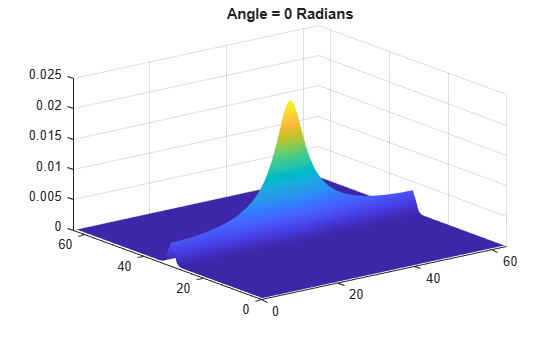Extract the wavelet corresponding to an angle of $\pi$/2 radians. Unlike the Mexican hat wavelet, the Morlet wavelet is not isotropic and therefore is sensitive to the direction of features in the data.

```surf(abs(cwtmorl.cfs(:,:,1,1,2))); shading interp; title('Angle = pi/2 Radians');```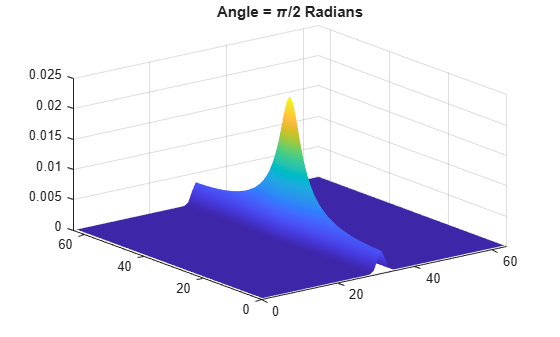Apply the Mexican hat and Morlet wavelets to the detection of a pattern in noise. Create a pattern consisting of line segments joined at a 90-degree angle. The amplitude of the pattern is 3 and it occurs in additive N(0,1) white Gaussian noise.

```X = zeros(256,256); X(100:200,100:102) = 3; X(200:202,100:125) = 3; X = X+randn(size(X)); imagesc(X); axis xy;```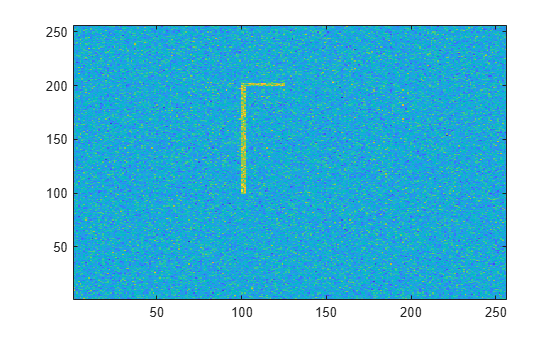Obtain the 2-D CWT at scales 3 to 8 in 0.5 increments with the Mexican hat wavelet. Visualize the magnitude-squared 2-D wavelet coefficients at scale 3.

```cwtmexh = cwtft2(X,'wavelet','mexh','scales',3:0.5:8); surf(abs(cwtmexh.cfs(:,:,1,3,1)).^2); view(0,90); shading interp; axis tight;```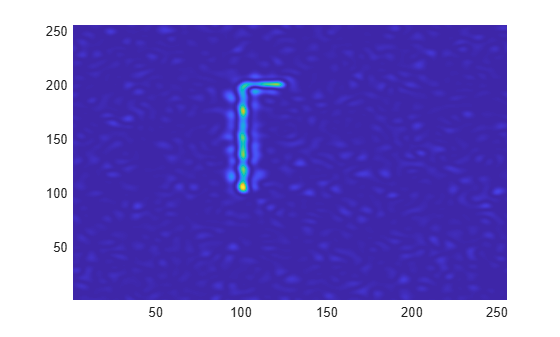Use a directional Morlet wavelet to extract the vertical and horizontal line segments separately. The vertical line segment is extracted by one angle. The horizontal line segment is extracted by another angle.

```cwtmorl = cwtft2(X,'wavelet','morl','scales',3:0.5:8,... 'angles',[0 pi/2]); surf(abs(cwtmorl.cfs(:,:,1,4,1)).^2); view(0,90); shading interp; axis tight;```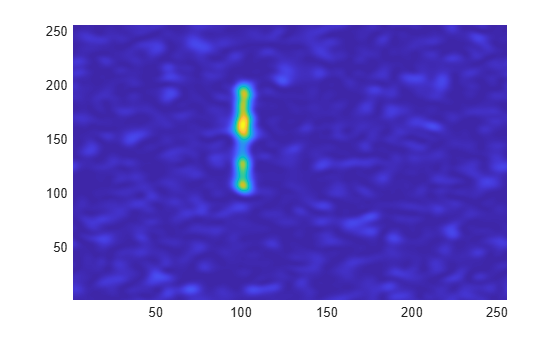```figure; surf(abs(cwtmorl.cfs(:,:,1,4,2)).^2); view(0,90); shading interp; axis tight;```